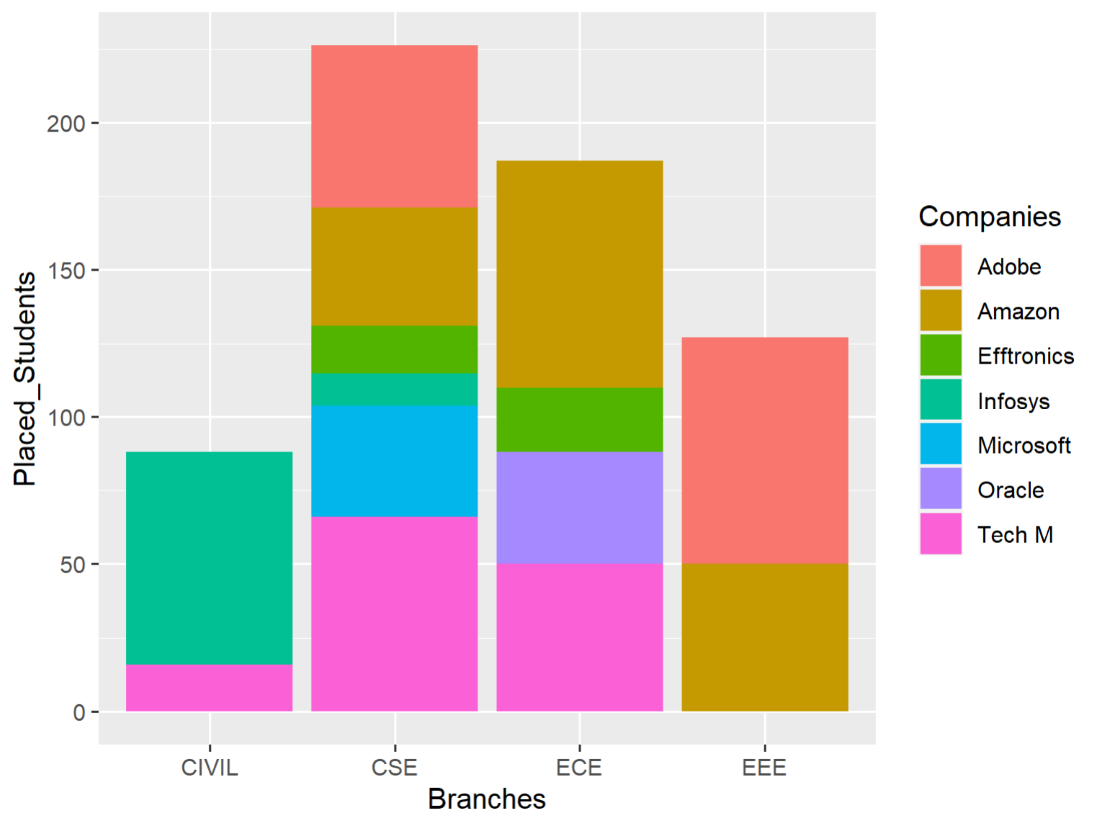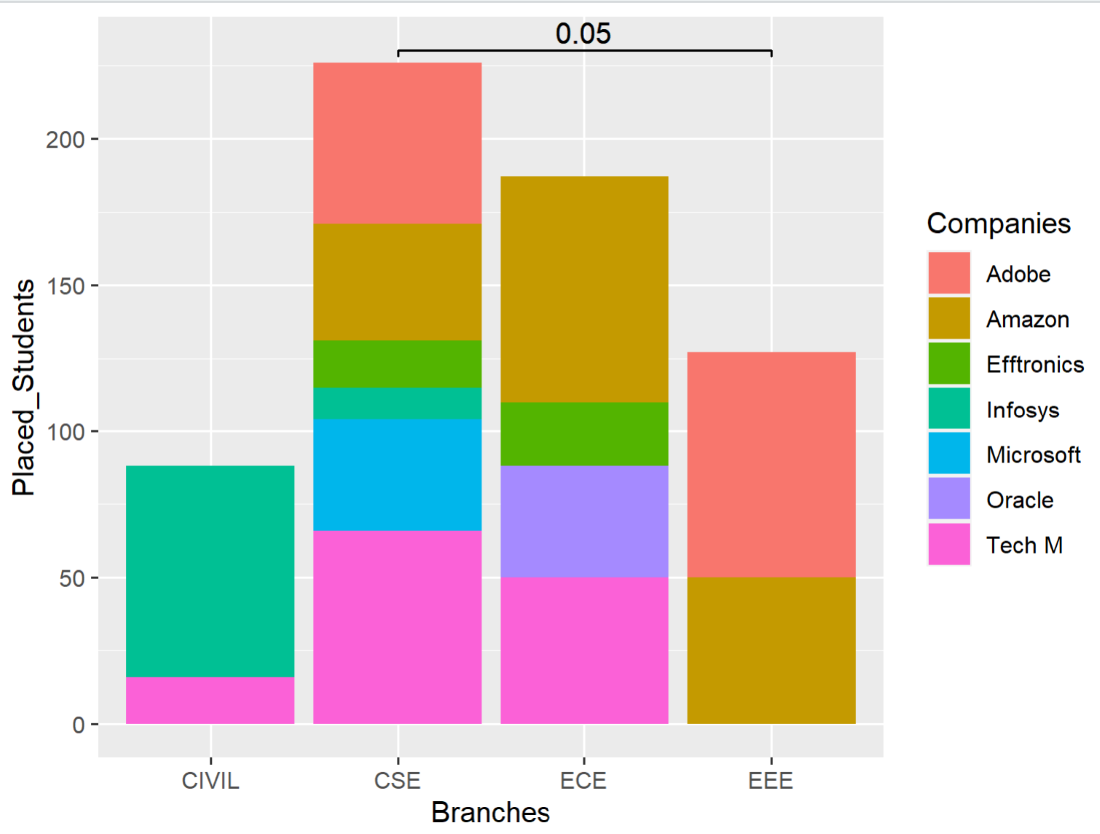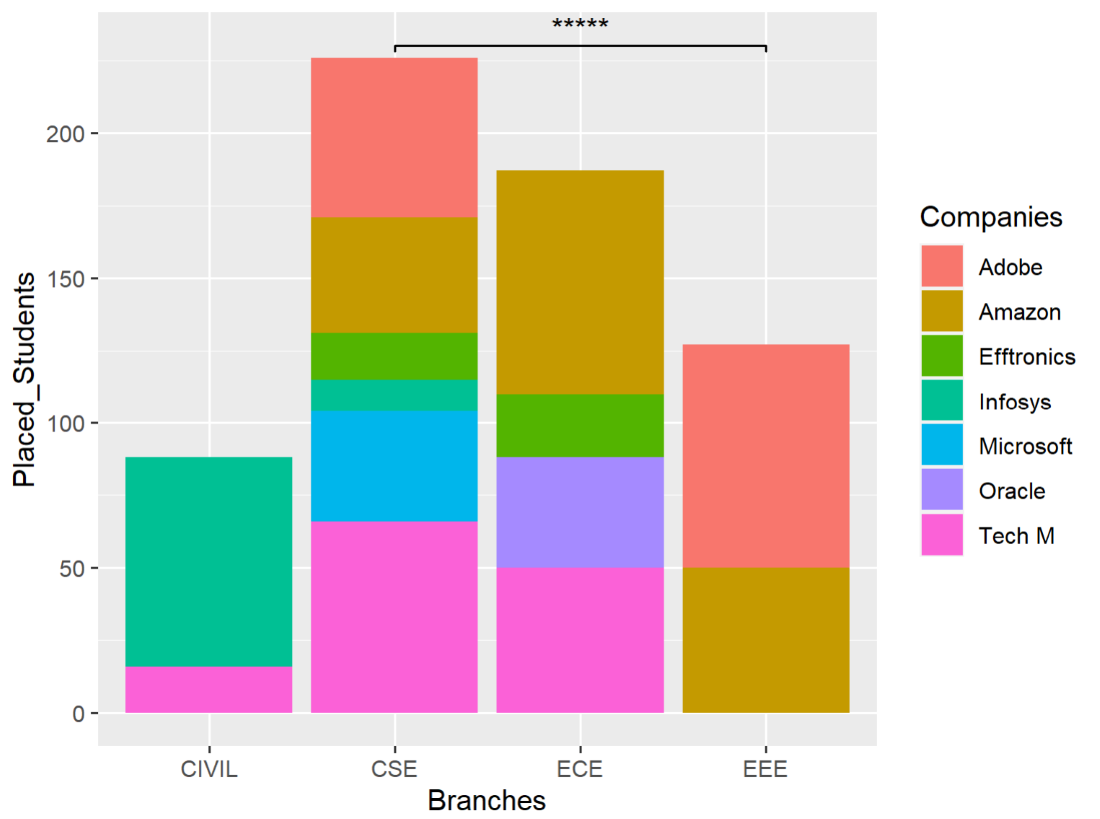Open in App
Not now

# Add Values to Stacked Bar Plot using ggsignif Package

• Last Updated : 07 Feb, 2023

The stacked bar plot is simply defined as the categorical plot which enables the user to plot one or more categorical values on the same bar. Stacking is the process of arranging one item on another. So Stacked Bar Plot is nothing but the stacking of two or categorical variables on the same plot. Let’s try to implement the same by using ggsignif package in the R programming Language. Install and load required packages.

## R

 `# install required packages``install.packages``(``"ggplot2"``)``install.packages``(``"ggsignif"``)` `# Load the installed packages``library``(ggplot2)``library``(ggsignif)`

Creation of a sample data frame with three categorical columns.

## R

 `# Creation of data frame``df <- ``data.frame``(Branches = ``c``(``"CSE"``, ``"CSE"``, ``"CSE"``, ``"CSE"``,``                              ``"ECE"``, ``"ECE"``, ``"EEE"``, ``"ECE"``,``                              ``"CSE"``, ``"CSE"``, ``"ECE"``, ``"CSE"``,``                              ``"CIVIL"``, ``"CIVIL"``, ``"EEE"``, ``"ECE"``),``                 ``Companies = ``c``(``"Amazon"``, ``"Microsoft"``, ``"Adobe"``,``                               ``"Infosys"``, ``"Oracle"``, ``"Amazon"``,``                               ``"Adobe"``, ``"Efftronics"``, ``"Efftronics"``,``                               ``"Tech M"``, ``"Amazon"``, ``"Adobe"``,``                               ``"Infosys"``, ``"Tech M"``, ``"Amazon"``, ``"Tech M"``),``                 ``Placed_Students = ``c``(40, 38, 44, 11, 38, 61,``                                     ``77, 22, 16, 66, 16, 11,``                                     ``72, 16, 50, 50)``                ``)`

Plotting the Stacked Bar Plot using ggplot2.

## R

 `# Plotting the stacked bar plot``p <- ``ggplot``(df, ``aes``(Branches,``                    ``Placed_Students,``                    ``fill = Companies)) +``       ``geom_col``()``p`

Output:Addition of P-Values to stacked Bar Plot. The P Values are used to display the significate between two categorical variables on the plot using the geom_signif() of ggsignigf package.

Syntax:

geom_signif(data, stat, position, comparisons, map_signif_level)

Where,

• data – The data need to be displayed on the layer.
• stat – The statistical transformation used on the data(“signif”)
• position – The string or function to adjust the position(“identity”)
• comparisons – A list of length-2 vectors where the significance level need to be compared.
• map_signif_level – Set to TRUE to display the default significance level annotation else FALSE.
• annotations – used to set some value which represents the significance level(like “***”,0.05 etc,.)

## R

 `# Adding P values to Bar Plot using ggsignif``p <- p + ggsignif::``geom_signif``(annotations = 0.05,``                               ``y_position = 230,``                               ``comparisons=``list``(``c``(``'CSE'``,``'EEE'``)))``p`

Output:Adding Stars to the Stacked Bar Plot using ggsignif.

## R

 `#Adding Stars to the Plot``p <- ``ggplot``(df, ``aes``(Branches, Placed_Students,``                    ``fill = Companies)) +``     ``geom_col``() +``     ``geom_signif``(annotations=``"*****"``,``                 ``y_position = 230,``                 ``xmin=``"CSE"``, xmax=``"EEE"``)``p`

Output:My Personal Notes arrow_drop_up